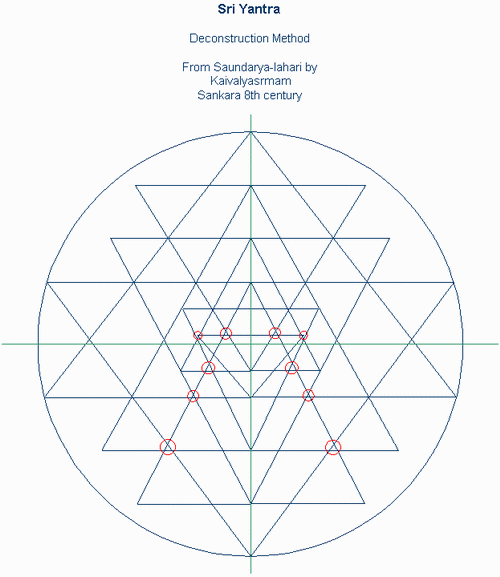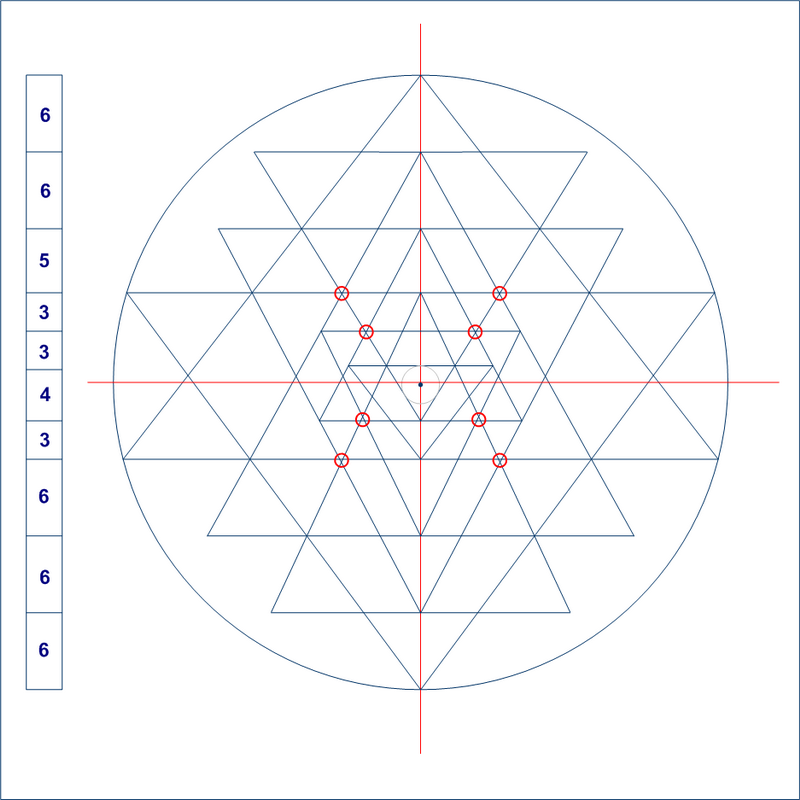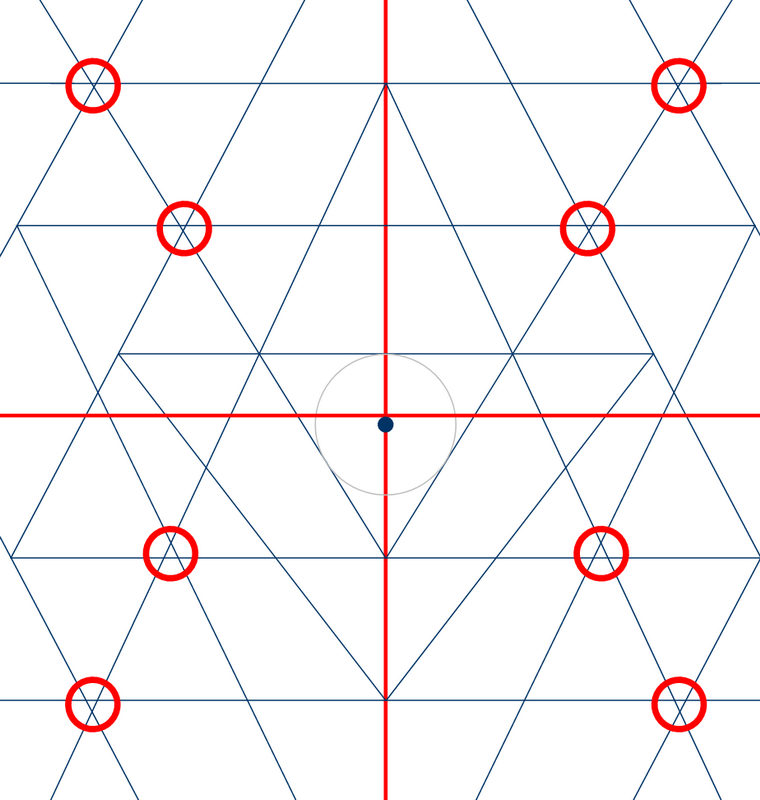Classic Construction Methods

 1. Inward construction method from commentary on Saundarya-lahiri written by Kaivalyashrama.
 This is the figure we obtain if we apply the instructions that can be found here. 1. Draw a circle of the required size with a vertical line through the centre and divide this line into 48 equal units. 2. On this line make nine marks at a distance of 6, 12, 17, 20, 23, 27, 30, 36, and 42 units from the top, and draw nine horizontal lines (numbered 1-9) through these marks to meet with the circle. 3. At both ends of the 1st, 2nd, 4th, 5th, 6th, 8th, and 9th lines rub off 3, 5, 16, 18, 16, 4 and 3 units respectively. 4. Join the ends of the 1st line to the centre of the 6th, the ends of the 2nd to the centre of the 9th, the ends of the 3rd to the circle at the bottom of the axis, the ends of the 4th to the centre of the 8th, the ends of the 5th to the centre of the 7th, the ends of the 6th to the centre of the 2nd, the ends of the 7th to the circle at the top of the vertical axis, the ends of the 8th to the centre of the 1st, and the ends of the 9th to the centre of the 3rd.After applying this method we obtain a very crude aproximation with errors on many of the triple junction points. The reason for so many errors is a result of not following the proper drawing sequence shown here. Another version of this method can be found here. By applying this variation we obtain the following figure:Again this mehod produces more errors than necessary at the triple intersections. Here is a detailed view of the center area.We also notice that the figure is not concentric, i.e. the center of the circle doesn't match with the center of the inner most triangle. One could reduce the number of errors by applying the proper drawing sequence to this method.